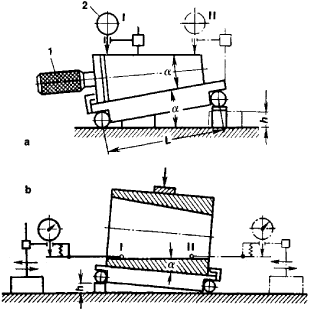# Sine Bar

Also found in: Wikipedia.

## sine bar

[′sīn ‚bär]
(design engineering)
A device consisting of a steel straight edge with two cylinders of equal diameter attached near the ends with their centers equidistant from the straightedge; used to measure angles accurately and to lay out work at a desired angle in relationship to a surface.
McGraw-Hill Dictionary of Scientific & Technical Terms, 6E, Copyright © 2003 by The McGraw-Hill Companies, Inc.
The following article is from The Great Soviet Encyclopedia (1979). It might be outdated or ideologically biased.

## Sine Bar

a special ruler in the form of a right parallelepiped with a cylindrical roller or sphere at each of the two ends. Sine bars are used to compute angles in the manufacture or measurement of parts, for example, cones and wedges.

In operation, a gage block is placed under one roller of the base of the sine bar. The height of the gage block h is calculated according to the formula h = L sin α, where a is the angle at which the plane of the sine bar must lie and L is the distance between the axes of the rollers. The distance between the rollers is usually 100 to 500 mm, and the angle is set in one direction or in two mutually perpendicular directions. The part being measured or worked is placed on the flat surface of the sine bar or in centers. Sine bars are most often used together with a dial gauge for determining the angle error of taper gauges (see Figure 1). The sine bar is adjusted to the nominal angle of the taper; the deviation from the horizontal formed by the taper relative to the base of the sine bar is read from the dial gauge.Figure 1. Angle measurement using a sine bar: (a) external taper, (b) internal taper; (1) taper gauge, (2) dial gauge; (I) and (II) positions of the dial gauge

Sine bars are usually used to set angles from 0° to 45° with an error from 4″ to 15″, depending on the nominal distance between the rollers, the magnitude of the angle at which the sine bar is set, and the precision of the construction of the sine bar. The principle of the sine bar is used, for example, in the design of various instruments for the transmission of motion at an angle to the primary motion and in attachments for metal-cutting machines used to work parts with inclined surfaces.

### REFERENCES

Eidinov, V. Ia. Izmerenie uglov v mashinostroenii. Moscow, 1963.
Konicheskie soedineniia. Moscow, 1968.

N. N. MARKOV [23–1295–]